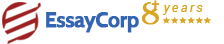WhatsApp
Struggling With Assignments?

We are here to help.

Chat with our expert writers on WhatsApp

for any kind of assignment queries.

Click to chat!
Struggling with Assignments? WhatsApp Us## Question:

Three particles A, B and C lie in that order in a straight smooth groove so that they can only move along the x-axis. Both A and C are of unit mass whilst B is of mass M. Particle B is joined to the other two by identical linear springs of unit stiffness.

1. If the three particles are displaced from equilibrium by distances x1, x2 and x3 show that the equations of motion are
• d2x1/ dt2 = x2 - x1,
• M d2x2/dt2 = x3 - 2x2 + x1
• d2x3/dt2 = x2 - x3.
2. Prove that the frequencies of the three normal modes are given by ? = 0, 1 and ?2 = 1 + (2/M). [5 marks]
3. Describe the motions of the particles corresponding to the normal modes.[4 marks]

(a) The equations of displacement for this system are generally:Which only serve for n=2 for said system as they are. For the other two cases, we haveSince ki=1 for all springs and m2=M, m1,3=1 , we get(b) The solutions of the displacement equations are. Substituting these solutions to the equations derived in a), we get the following system:The eigenvalues of which are derived by setting the determinant equal to 0. This way, we obtain the equationThe solutions of which are(c) Plugging the eigenvalues for ω in the system, we getFor ω=0, the first and the second row give u1 = u2 = u3 , but since there is no frequency , the bodies are at rest. For ω=1, the solutions are u1 = -u3, u2=0. This means that the body B does not move, but the other two oscillate around it, in opposite directions every time. Finally, forWhere all bodies oscillate, but the direction of the oscillation depends on the mass M of the second body.

• If M < 1 , u1 and u3 oscillate in the opposite directions, and u2 oscillates in the same direction with u1.
• If 1 > M >2, u1 and u3 oscillate in the opposite directions, and oscillates in the same direction with u3.
• If M > 2, u1 and u3 oscillate in the same directions, and u2 oscillates in the opposite direction with both of them.
HIRE EXPERT
Email ID *
Subject
Pages